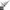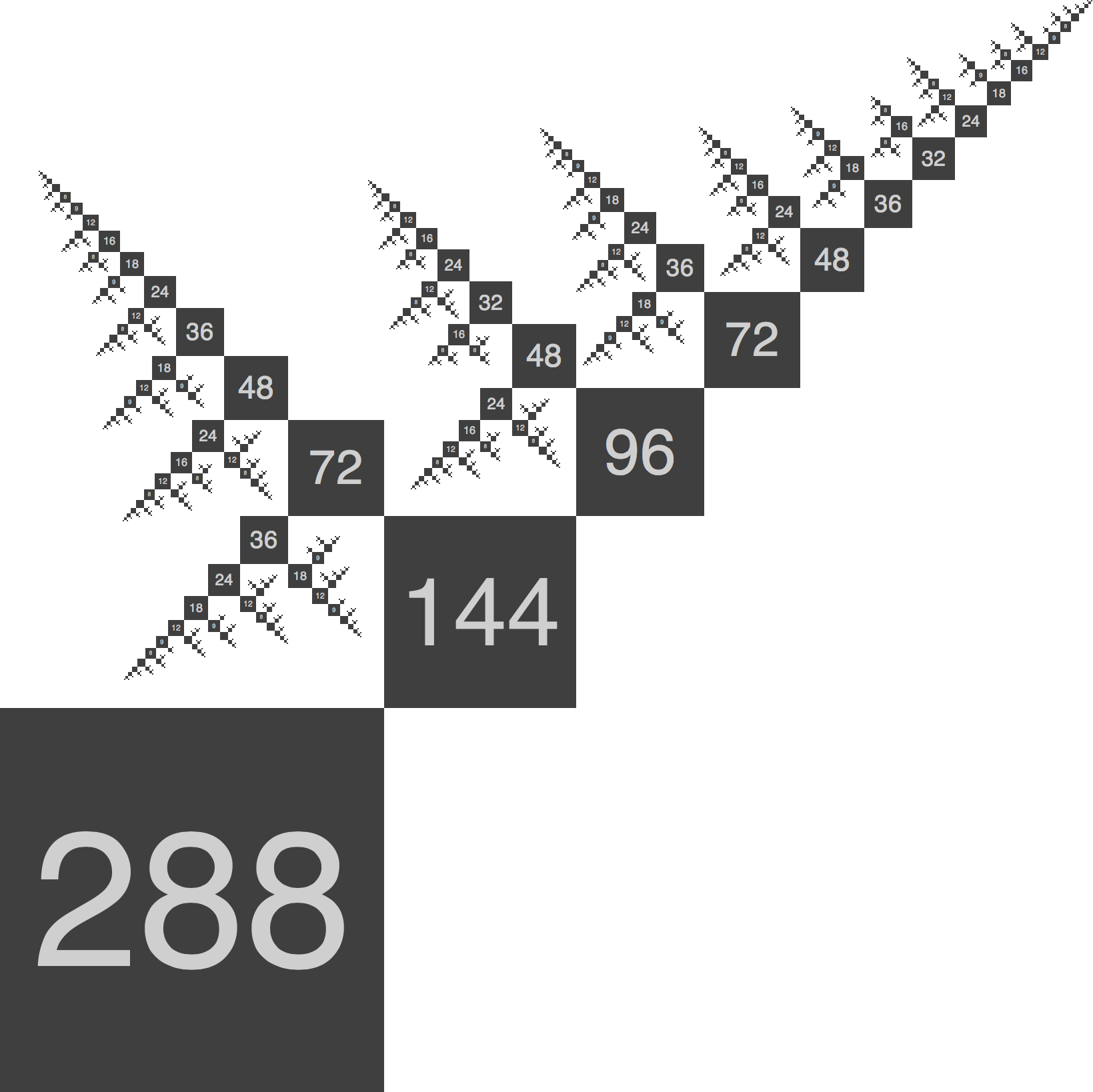# Recursively divisible numbers

Recursively divisible numbers are a new kind of number that are highly divisible, whose quotients are highly divisible, and so on.

T. FinkDivisible numbers are useful whenever a whole needs to be divided into equal parts. But sometimes it is necessary to divide the parts into subparts, and subparts into sub-subparts, and so on, in a recursive way. To understand numbers that are recursively divisible, I introduce the recursive divisor function: a recursive analog of the usual divisor function. I give a geometric interpretation of recursively divisible numbers and study their general properties in particular the number and sum of the recursive divisors. I show that the number of the recursive divisors is equal to twice the number of ordered factorizations into integers greater than one, a problem that has been much studied–in its own right. By computing those numbers which are more recursively divisible than all of their predecessors, I recover many of the grid sizes commonly used in graphic design and digital technologies, and suggest new grid sizes which have not yet been adopted but should be. These are especially relevant to recursively modular systems which must operate across multiple organizational length scales.T. Fink

1 / 123 papers